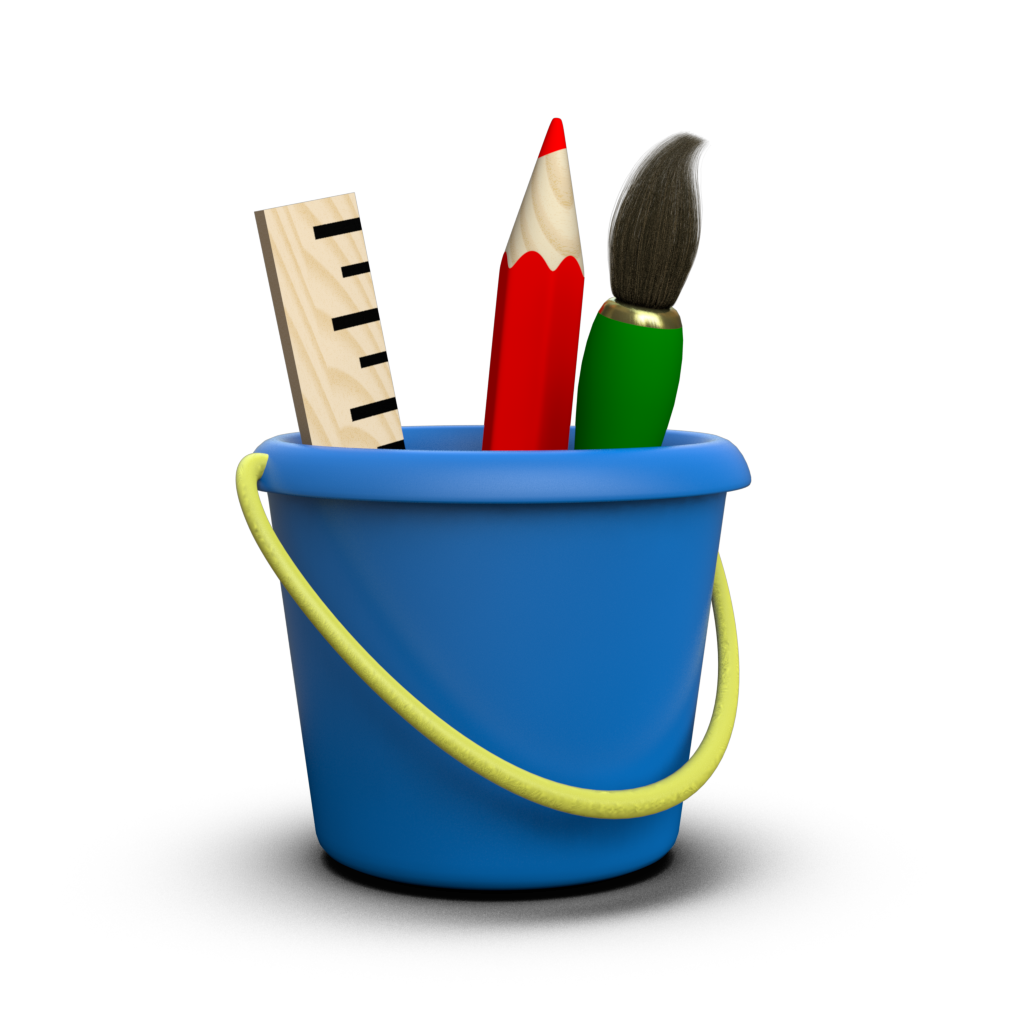# Canvas Layout¶

In Toyplot, coordinate systems (including Cartesian Coordinates, Numberline Coordinates, Table Coordinates, and others) are used to map data values into canvas coordinates. The coordinate system ranges (the area on the canvas that they occupy) is specified when they are created. By default, cartesian and table coordinates are sized to fill the entire canvas:

:

import numpy
y = numpy.linspace(0, 1, 20) ** 2

:

import toyplot
toyplot.plot(y, width=300);


If you need greater control over their positioning within the canvas, or want to add multiple coordinate systems to one canvas, it’s necessary to create the canvas and coordinate systems explicitly, then use the coordinate systems to plot your data. For example, you can use the bounds argument to specify explicit (xmin, xmax, ymin, ymax) bounds for cartesian axes using canvas coordinates (note that canvas coordinates always increase from top to bottom, unlike cartesian coordinates):

:

canvas = toyplot.Canvas(width=600, height=300)
axes1 = canvas.cartesian(bounds=(30, 270, 30, 270))
axes1.plot(y)
axes2 = canvas.cartesian(bounds=(330, 570, 30, 270))
axes2.plot(1 - y);


You can also use negative values to specify values relative to the right and bottom sides of the canvas, instead of the (default) left and top sides, greatly simplifying the layout:

:

canvas = toyplot.Canvas(width=600, height=300)
axes1 = canvas.cartesian(bounds=(30, 270, 30, -30))
axes1.plot(y)
axes2 = canvas.cartesian(bounds=(-270, -30, 30, -30))
axes2.plot(1 - y);


Furthermore, the bounds parameters can use any Units, including “%” units, so you can use real-world units and relative dimensioning in any combination that makes sense:

:

canvas = toyplot.Canvas(width="20cm", height="2in")
axes1 = canvas.cartesian(bounds=("1cm", "5cm", "10%", "80%"))
axes1.plot(y)
axes2 = canvas.cartesian(bounds=("6cm", "-1cm", "10%", "80%"))
axes2.plot(1 - y);


Of course, most of the time this level of control isn’t necessary. Instead, the grid argument allows us to easily position each set of axes on a regular grid that covers the canvas. Note that you can control the axes position on the grid in a variety of ways:

• (rows, columns, n)
• fill cell $$n$$ (in left-to-right, top-to-bottom order) of an $$M \times N$$ grid.
• (rows, columns, i, j)
• fill cell $$i,j$$ of an $$M \times N$$ grid.
• (rows, columns, i, rowspan, j, colspan)
• fill cells $$[i, i + rowspan), [j, j + colspan)$$ of an $$M \times N$$ grid.
:

canvas = toyplot.Canvas(width=600, height=300)
axes1 = canvas.cartesian(grid=(1, 2, 0))
axes1.plot(y)
axes2 = canvas.cartesian(grid=(1, 2, 1))
axes2.plot(1 - y);


You can also use the margin argument to control the space between cells in the grid:

:

canvas = toyplot.Canvas(width=600, height=300)
axes1 = canvas.cartesian(grid=(1, 2, 0), margin=25)
axes1.plot(y)
axes2 = canvas.cartesian(grid=(1, 2, 1), margin=25)
axes2.plot(1 - y);


Sometimes, particularly when embedding axes to produce a figure-within-a-figure, the corner argument can be used to position axes relative to one of eight “corner” positions within the canvas. The corner argument takes a (position, inset, width, height) tuple:

:

x = numpy.random.normal(size=100)
y = numpy.random.normal(size=100)

:

canvas = toyplot.Canvas(width="5in")
canvas.cartesian().plot(numpy.linspace(0, 1) ** 0.5)
canvas.cartesian(corner=("bottom-right", "1in", "1.5in", "1.5in")).scatterplot(x, y);


Here are all the positions supported by the corner argument:

:

canvas = toyplot.Canvas(width="12cm")
for position in [
"top-left",
"top",
"top-right",
"right",
"bottom-right",
"bottom",
"bottom-left",
"left",
]:
canvas.cartesian(corner=(position, "1cm", "2cm", "2cm"), label=position)